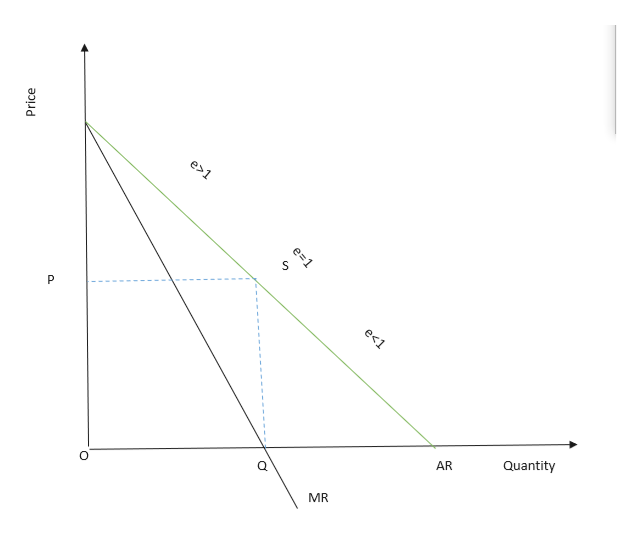Why does the monopolist always produce only until that level of output where the own-price elasticity of demand is equal to unity ? Elucidate this either graphically or algebraically

Question

Why does the monopolist always produce only until that level of output where the own-price elasticity of demand is equal to unity ? Elucidate this either graphically or algebraically

Step 1

A monopolist tends to maximise its profit and produces the output up to that level at which MC = MR, where the main aim of the monopolist is to earn profits.

The average revenue and marginal revenue at a given quantity are related to each other though elasticity.

We derive the following formula with the given condition:

MR = P {1- (1/e)}

where e is the elasticity of demand, P is the Price

We know, AR = Price

Therefore,MR=Price (e-1/e)

Or Price=MR (e/e-1)

If the expression e-1/e will be less than unity, then Marginal Revenue will be less than price. The extent to which MR will be less than price depends upon the value of the fraction (e-1/e).

Step 2

Under, Perfect competition marginal cost associate prices with quantity to be produced and supplied. But in monopoly’s case price is higher than marginal cost and therefore it does not associate price and quantity to be produced .Thus the MC curve cannot function as supply curve .Therefore no...help_outlineImage Transcriptionclosee1 e<1 P Quantity AR MR Price e 1 fullscreen

Want to see the full answer?

See Solution

Want to see this answer and more?

Our solutions are written by experts, many with advanced degrees, and available 24/7

See Solution
Tagged in